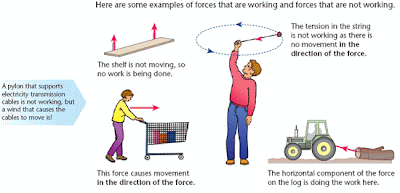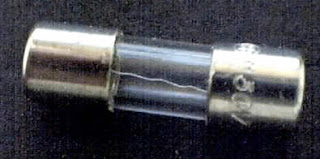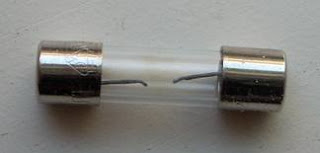## Wednesday, March 27, 2013

### What Is Electric Power?

If we are going to recall our Physics subject, it is said that whenever a force is applied that causes motion the work is said to be done. Take a look on the illustration below:
﻿Forces that work is done and  forces not doing work.
The first figure shown above are combination of forces which work is done and forces which work is not done. (a)The picture in which the shelf is held under tension does not cause motion, thus work is not done. (b) The second picture in which the woman pushes the cart causes motion, thus the work is done. (c) The man applied tension in the string is not working since as there is no movement in the direction of the force. (d) The track applied horizontal force on the log is doing work.

The potential difference between any two points in an electric circuit, which gives rise to a voltage and when connected causes electron to move and current to flow. This is one of a good example in which forces causing motion, thus causing work to be done.

Talking about work in electric circuit, there is also a electric power which is the time rate of doing work done of moving electrons from point to point. It is represented by the symbol P, and the unit of power is watt, which is usually represented by the symbol W. Watt is practically defined as the rate at which work is being done in a circuit in which the current of 1 ampere is flowing when the voltage applied is 1 volt.

The Useful Power Formula

Electric Power can be transmitted from place to place and can be converted into other forms of energy. One typical energy conversion of electrical energy are heat, light or mechanical energy. Energy conversion is what the engineers really mean for the word power.

The power or the rate of work done in moving electrons through a resistor in electric circuit depends on how many electrons are there to moved. It only means that, the power consumed in a resistor is determined by the voltage measured across it, multiplied by the current flowing through it. Then it becomes,

Power = Voltage x Current
Watts  = Volts x Amperes

P = E x I  or P = EI ------> formula no.1

The power formula above can be derived alternatively in other ways in terms of resistance and current or voltage and resistance using our concept of Ohm's Law. Since E=IR in Ohm's Law, the E in the power formula above can be replaced by IR if the voltage is unknown. Therefore, it would be:

P = EI
P = (IR)I or P = I2R ------------> formula no.2

Alternatively if I = E/R in Ohm'Law, we can also substitute it to E in the power formula which is terms of voltage if the resistance is unknown.

P = EI
P = E(E/R) or P = E2R ---------> formula no. 3

For guidance regarding expressing of units of power are the following:
a. Quantities of power greater than 1,000 watts are generally expressed in (kW).
b. Quantities greater than 1,000,000 watts are generally expressed as megawatts (MW).
c.  Quantities less than 1 watt are generally expressed in (mW).

The Power Rating of Equipment

Most of the electrical equipment are rated in terms of voltage and power - volts and watts. For example, electrical lamps rated as 120 volts which are for use in 120 volts line are also expressed in watts but mostly expressed in watts rather than voltage. Probably you would wonder what wattage rating all about.

The wattage rating of an electrical lamps or other electrical equipment indicates the rate at which electrical energy is changed into another form of energy, such as heat or light. It only means the greater the wattage of an electrical lamp for example, the faster the lamp changes electrical energy to light and the brighter the lamp will be.

The principle above also applies to other electrical equipment like electric soldering irons, electrical motors and resistors in which their wattage ratings are designed to change electrical energy into some forms of energy. You will learn more about other units like horsepower used for motors when we study motors.

Take a look at the sizes of carbon resistors below. Their sizes are depends on their wattage rating. They are available with same resistance value with different wattage value. When power is used in a material having resistance, electrical energy is changed into heat. When more power are used, the rate at which electrical energy changed into heat increases, thus temperature of the material rises. If the temperature of the material rises too high, the material may change it composition: expand, contract or even burn. In connection to this reason, all types of electrical equipment are rated for a maximum wattage.
﻿Carbon resistors with comparative sizes of different wattage ratings of 1/4 watt, 1/2 watt,1 and 2 watts
If the resistors greater then 2 watts rating are needed, wire-wound resistors are used. They are ranges between 5 and 200 watts, with special types being used for power in excess of 200 watts.

﻿Use wire wound resistors if higher than 2 watts are needed

Fuses

We all know that when current passes through the resistors, the electrical energy is transformed into heat which raises the temperature of the resistors. If the temperature rises too high, the resistor may be damaged thereby opening the circuit and interrupting the current flow. One answer for this is to install the fuse.

Fuses are resistors using special metals with very low resistance value and a low melting point. When the power consumed by the fuses raises the temperature of the metal too high, the metal melts and the fuse blows thus open the circuit when the current exceeds the fuse's rated value. What is the identification of blown fuse? Take a look on the picture below.

﻿This is the good fuse
﻿
﻿This is the blown fuse
﻿ In other words, blown fuses can be identified by broken filament and darkened glass. You can also check it by removing the fuse and using the ohmmeter.

There are two types of fuses in use today - conventional fuses, which blow immediately when the circuit is overloaded. The slow-blowing (slo-blo) fuses accepts momentary overloads without blowing, but if the overload continues, it will open the circuit. This slo-blo fuses usually used on motors and other appliances with a circuit that have a sudden rush of high currents when turned on.

Fuses are rated in terms of current. Since various types of equipments use different currents, fuses are also made with different sizes, shapes and current ratings.Various types of fuses are made for various equipments
Proper rating of fuse is needed and very important. It should be slightly higher than the greatest current you expect in the circuit because too low current rating of fuse will result to unnecessary blowouts while too high may result to dangerously high current to pass.

Later we will be study circuit breaker which is another protective devices for over current protection.

Electrical Power in Series, Parallel and Complex Circuits

The principle of getting the total power of the circuit is just simple. There is no need to elaborate this topic.

The total power consumed by the circuit is the sum of all power consumed in each resistance.

Therefore, we just only sum up all power consumed in each resistance whether it a series, parallel or a complex circuits. Thus,

Pt= P1+P2+P3+Pn watts  ---------->formula no. 4

From the problem in my previous post about complex circuit, try to calculate each power of the resistance and the total power as well. Constant practice always makes you perfect!